﻿ Thermal Effect on Vibration of Orthotropic Rectangular Plate with Thickness Variation as Linearly & Parabolically in x- and y- Directions Respectively

### Thermal Effect on Vibration of Orthotropic Rectangular Plate with Thickness Variation as Linearly &a...

Arun Kumar Gupta, Subodh KumarOPEN ACCESSPEER-REVIEWED

## Thermal Effect on Vibration of Orthotropic Rectangular Plate with Thickness Variation as Linearly & Parabolically in x- and y- Directions Respectively

Arun Kumar Gupta1,, Subodh Kumar2

1Department of Mathematics, M. S. College, Saharanpur, U.P., India

2Department of Mathematics, Govt.College, Ambala Cantt, Haryana, India

### Abstract

The main objective of the present investigation is to study linear temperature behavior of orthotropic rectangular plate of bi-direction variable thickness. The thickness of the plate is considered as linearly in x-direction and parabolically in y-direction. Rayleigh-Ritz technique has been employed to obtain fundamental frequencies for the first two modes of vibrations. The effect of structural parameters such as taper constants and thermal gradient with c-c-c-c boundary condition has been taken. Numerical results thus obtained are shown graphically. Excellent agreement is noted when comparison is made with previous available experimental data.

### At a glance: Figures

12
Prev Next

• Gupta, Arun Kumar, and Subodh Kumar. "Thermal Effect on Vibration of Orthotropic Rectangular Plate with Thickness Variation as Linearly & Parabolically in x- and y- Directions Respectively." Journal of Mathematical Sciences and Applications 1.2 (2013): 32-35.
• Gupta, A. K. , & Kumar, S. (2013). Thermal Effect on Vibration of Orthotropic Rectangular Plate with Thickness Variation as Linearly & Parabolically in x- and y- Directions Respectively. Journal of Mathematical Sciences and Applications, 1(2), 32-35.
• Gupta, Arun Kumar, and Subodh Kumar. "Thermal Effect on Vibration of Orthotropic Rectangular Plate with Thickness Variation as Linearly & Parabolically in x- and y- Directions Respectively." Journal of Mathematical Sciences and Applications 1, no. 2 (2013): 32-35.

 Import into BibTeX Import into EndNote Import into RefMan Import into RefWorks

### 1. Introduction

In modern technology an interest towards the effect of high temperatures on elastic plates of variable thickness is developed due to applications in various engineering branches such as nuclear, power plants, aeronautical, chemical etc. where metals and their alloys exhibits elastic behavior. Therefore for these changes the structures are exposed to high intensity, heat fluxes and material properties undergo significant changes.

Tapered plates are used quite often to model structures used in a wide variety of applications. Vibration problems of elastic plate is very much comprehensive due to various geometrical shapes with complications of anisotropy, visco-elastic, non-homogeneity, variable thickness, surrounding media, in plane force, large deflections elastic foundation, shear deformation and rotatory inertia, simple and mixed boundary conditions.

The materials are being developed, depending upon the requirement and durability, so that these can be used to give better strength, flexibility, weight effectiveness and efficiency. So some new materials and alloys are utilized in making structural parts of equipment used in modern technological industries like space craft, jet engine, earth quake resistance structures, telephone industry etc. Applications of such materials are due to reduction of weight and size, low expenses and enhancement in effectiveness and strength. It is well known that first few frequencies of structure should be known before finalizing the design of a structure. The study of vibration of plate structures is important in a wide variety of applications in engineering design. Elastic plates are widely employed nowadays in civil, aeronautical and marine structures designs. Complex shapes with variety of thickness variation are sometimes incorporated to reduce costly material, lighten the loads, and provide ventilation and to alter the resonant frequencies of the structures.

Leissa’s monographs  provided a sufficient amount of study up to 1985 for various types of thickness variations and edge conditions. Studies on different types of plates such as circular, elliptical and rectangular have been carried out by a number of researchers. Laura and Gutierrez  discussed vibration analysis of a rectangular plate subjected to a thermal gradient. Yang  studied the vibrations of a circular plate with variable thickness. Gupta and Khanna  solved the problem of vibrations of clamped visco-elastic rectangular plate with parabolic variable thickness. Shibaoka  studied transverse vibration of elliptic plate with clamped edge. Effect of thermal gradient on free vibration of clamped visco elastic plate was discussed by Gupta and Kaur . Gupta and Kumar  studied the thermal effect on vibration of orthotropic rectangular plate with parabolic thickness variations. Chen et al.  worked on free vibration of non-homogeneous transversely isotropic magneto-electro-elastic plate. Pan and Heyliger  evaluated the free vibrations of simply supported and multilayered magneto-electro-elastic plates. Chakarverty et al.  studied the flexural vibrations of non-homogeneous elliptic plates. Tomar and Gupta [11, 12, 13] solved the vibration problem of orthotropic rectangular plate of varying thickness subjected to a thermal gradient.

Gupta and Khanna  have studied the effect of linearly varying thickness in both directions on vibration of visco-elastic rectangular plate. Gupta and Kaur  studied the effect of thermal on vibration of clamped visco-elastic rectangular plate with linearly thickness in both directions. Gupta, Kumar and Gupta  have considered the vibration of visco-elastic parallelogram plate with parabolic thickness variation. Gupta and Singhal  discussed the effect of non-homogeneity on thermally induced vibration of orthotropic visco-elastic rectangular plate of linearly varying thickness.

The main object of the present study is to determine the thermal effect on vibration of orthotropic rectangular plate with bi-direction varying thickness having clamped boundary conditions on all the four edges. Rayleigh Ritz method is used to evaluate the frequency parameter. The two-dimensional thickness variation is taken as the Cartesian product of linear and parabolic variations respectively along the two concurrent edges of the plate. Yet the method used for the solution of present problem provides an approximate solution but it is quite convenient and authentic one. A two-term deflection function is used. Frequency parameter is calculated for first two modes of vibration for clamped orthotropic rectangular plate, whose thickness varies linearly in one direction and parabolically in another & is subjected to linearly varying temperature distribution, for various values of thermal gradient α and taper constants β1, β2. These results have been compared with those obtained for orthotropic rectangular plate with bi-directional linear and parabolic variation in thickness respectively with linearly varying temperature distribution in one direction.

### 2. Equations of Motion

When the plate is executing transverse vibration of mode shape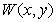,then Strain energy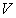and Kinetic energy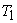are respectively .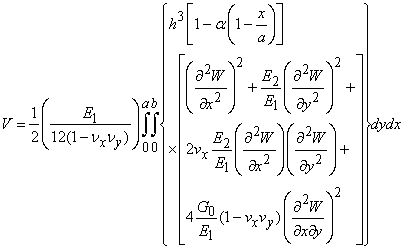(1)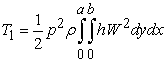(2)

### 3. Variation in Thickness

Thickness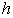of the plate is assumed to be varying linearly in one direction and parabolic in another, i.e.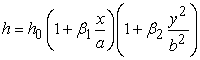(3)

where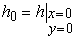.

Using equation (3) in equations (1) & (2), one has,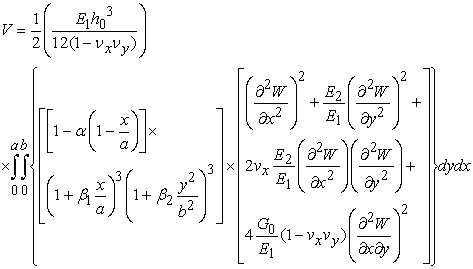(4)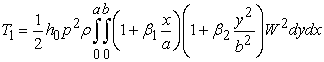(5)

### 4. Method of Solution

An approximate solution to the current problem is given by the application of Rayleigh – Ritz method. In order to apply their procedure, maximum Strain energy must be equal to maximum Kinetic energy. Therefore it is desired that following equation must be satisfied: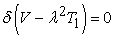(6)

On substituting the values of ‘V’ & ‘T1’ from equations (4) & (5) in equation (6), one has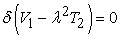(7)

where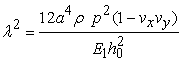(8)

is a frequency parameter,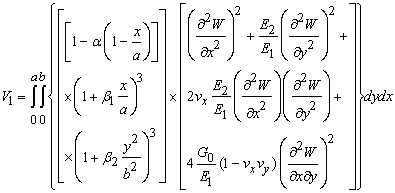(9)

and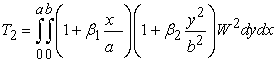(10)

### 5. Boundary Condition and Frequency Equatio

For a clamped rectangular plate, boundary conditions are,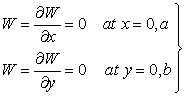(11)

Equation (7) contains two unknown parameters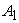&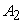to be evaluated. Values of these constants may be evaluated by the following procedure: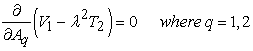(12)

On simplifying equation (12), we get following form,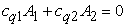(13)

where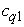&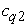involves the parametric constants and the frequency parameter.

For a non - zero solution, determinant of coefficients of equation (13) must vanish. In this way frequency equation comes out to be,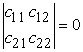(14)

### 6. Results and Discussion

Frequency parameter is calculated for the first two modes of vibration for different values of taper constants and thermal gradient, for a clamped plate. Frequency equation (14) is quadratic in λ2, so will give two roots. Let they be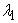&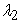respectively. These two values correspond to first and second modes of vibration respectively.

The results have been explained with the help of figures and plotted between various parameters shown in Figures 1 to 5.

The parameter for orthotropic material has been taken as,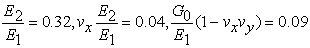Verification of work can be done by comparing these with the problems discussed  or  by allowing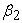or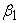equal to zero respectively. On makingequal to zero in present problem the problem reduces to that of discussed in chapter-1, if we allowbeing zero in that problem too. It was found that on doing so same results are coming and hence verified the present work. In a similar way if we allowequals to zero then we can compare these results with those materialized in  providedis also zero there. The results provide evidence to the authenticity of work.

Figure 1. VARIATION OF FREQUENCY PARAMETER λ2 FOR DIFFERENT VALUES OF THERMAL GRADIENT α

Figure 1 shows variation of frequency parameter ‘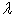’ with thermal gradient parameter ‘α’, for various values of taper constants&, for a clamped plate for both modes of vibration. From Figure 1 it is clear that with increase in ‘α’, ‘’ decrease whether&are zero or non-zero. It is to be noticed that ‘’ decreases sharply for second mode of vibration as compared to the first mode of vibration.

Figure 2 and Figure 3 display the variation of taper constant ‘β1’ with frequency parameter ‘’, for both modes of vibration. It is observed that for both the modes of vibration ‘’ increases with increase in ‘β1’, whether the plate is heated or unheated. For non-zero ‘β2’, ‘’ has higher value and from unheated to heated plate, value of ‘’ decrease. Similar pattern is found in case of ‘’ referring to Figure 4 and Figure 5. If a comparison is made between ‘’ & ‘’ it is found that in case of ‘’, ‘’ has larger values.

Figure 5. VARIATION OF FREQUENCY PARAMETER λ2 FOR DIFFERENT VALUES OF TAPER CONSTANT β2

A comparative study was done for the plates regarding variation in thickness i.e. plates with linear and parabolic variations in thickness were compared with the present kind of variation in thickness. In present problem thickness variation is linear in x-direction and parabolic in y-direction. On comparing this with linear variation in thickness, it is found that all the effects are occurring in the similar manner but for lesser values for the current problem. Hence as compared to linear variation in thickness, present kind of variation is better.

On comparing the same problem with parabolic variation in thickness, it was realized that parabolic variation in thickness is much better as compared to this kind of variation in thickness. Hence it is concluded that plates with parabolic variation in thickness are more stable as compared to those of linearly varying thickness, and to the present kind of problem for bearing up of thermally induced vibration effects.

### References

  A. W. Leissa: Vibration of plates. NASA-SP-160, U.S. Govt. Printing Office, (1969).In article  P. A. A. Laura, R. H. Gutierrez: Vibration analysis on a rectangular plate subjected to a thermal gradient. J. Sound and Vibration, 72 (1980), 263.In article CrossRef  Y. S. Yang: The vibrations of a circular plate with variable thickness. J. Sound and Vibration, 165 (1993), 178-184.In article CrossRef  A. K. Gupta, A. Khanna: Vibration of clamped visco-elastic rectangular plate with parabolic thickness variations. Shock and Vibration, 15 (2008), 713-723.In article  Y. Shibaoka: On the transverse vibration of an elliptic plate with clamped edge. J. Phy. Soc. Japan, 11 (1956), 797-803.In article CrossRef  A. K. Gupta, H. Kaur: Study of the effect of thermal gradient on free vibration of clamped visco-elastic rectangular plates with linearly thickness variation in both directions. Meccanica, 43 (2008), 449-458.In article CrossRef  A. K. Gupta, S. Kumar: Thermal effect on vibration of orthotropic rectangular plate with parabolic thickness variations. Acta Technica, 55 (2010), 315-325.In article  W. Q. Chen, K. Y. Lee, H. J. Ding: On free vibration of non-homogeneous transversely isotropic magneto-electro-elastic plate. J. Sound and Vibration, 279 (2005), 237-251.In article CrossRef  E. Pan, P. R. Heyliger: Free vibrations of simply supported and multilayered magneto-electro-elastic plates. J. Sound and Vibration, 252 (2002), 429-442.In article CrossRef  S. Chakraverty, R. Jindal, V. K. Agarwal: Flexural vibrations of non-homogeneous elliptic plates. Indian J. Engineering and Materials Sciences, 12 (2005), 521-528.In article  J.S. Tomar and A.K.Gupta: Effect of thermal gradient on frequencies of orthotropic rectangular plate whose thickness varies in two directions, J.Sound and Vibration, 98(1985), 257-262.In article CrossRef  J.S. Tomar and A.K.Gupta: Thermal effect on frequencies of an orthotropic rectangular plate of linearly varying thickness, J.Sound and Vibration,90(1983), 325-331.In article CrossRef  J.S. Tomar and A.K.Gupta: Effect of exponential temperature variation on frequencies of an orthotropic rectangular plate of exponentially varying thickness, Proceeding of the workshop on computer application in continuum mechanics, March 11-13(1988), Deptt. of Math. U.O.R, Roorkee, 183-188.In article  A.K. Gupta and A.Khanna: Vibration of visco-elastic rectangular plate with linearly thickness variations in both directions, J. Sound and Vibration, 301(2007), 450-457.In article CrossRef  A.K.Gupta and H.Kaur: Study of the effect of thermal gradient on free vibration of clamped visco-elastic rectangular plate with linearly thickness variation in both directions, Meccanica, 43(2008), 449-458.In article CrossRef  A.K.Gupta, A. Kumar and Y.K.Gupta : Vibration of visco-elastic parallelogram plate with parabolic thickness variation, Applied Mathematics, 1(2010), 128-136.In article CrossRef  A.K. Gupta and P. Singhal: Effect of non-homogeneity on thermally induced vibration of orthotropic visco-elastic rectangular plate of linearly varying thickness, Applied Mathematics, 1(2010), 326-333.In article CrossRef